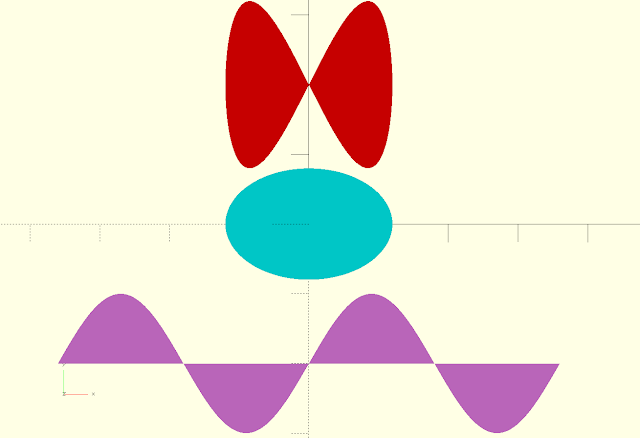## Polygon and polyhedron

OpenSCAD allows the user to create complex shapes with the polygon function for 2D and polyhedron for 3D. Polygon and polyhedron both accept a list of 2D and 3D coordinates (points) respectively as parameters. A functions can generate a list of points eliminating the need to manually created these lists. This property can be used to create shapes that are impossible with the 2D and 3D shapes that are build-in in OpenSCAD. In this blog post I'll show how to create functions for some simple 2D shapes and explain how to manipulate the functions make more complex shapes with them.Shapes created with functions and polygon in OpenSCAD.

## Creating a 2D shape

To create a circle with a radius of 20 in OpenSCAD we just have to type

circle(20);

However OpenSCAD doesn't allow us to reshape this build-in function to for instance an ellipse. Alternatively we can write a function that generates a list of points needed for a circle and then use polygon with the points as parameter to draw the circle. The function uses the trigonometric formulas, x = r cos φ and y = sin φ, to convert polar coordinates to Cartesian coordinates.

function circle(radius) = [for (phi = [1 : 1 : 360]) [radius * cos(phi), radius * sin(phi)]];
polygon(circle(20));

When F5 is pressed a circle is drawn however the x,y coordinates of this circle are available to us. By adding echo(circle(20)); to our script the list of points is printed in the console. The circle function can easily be altered thus gaining a new shape. An example is shown below.

function circle(radius) = [for (phi = [0 : 1 : 720]) [radius * cos(phi/2), radius * sin(phi)]];
color("red") polygon(circle(20));Shape create with x = r cos(φ/2) and y = r sin(φ)

Now let's take a look at the syntax of the function. Every function generates a value and in this case it is a list of points. In OpenSCAD a list of points in a two-dimensional space is represented by [[x1,y1],[x2,y2],[x3,y3],...] where all x's and y's are numbers. In this case of the circle function the point are generated in a for loop. The loop begin at 0 and ends at 720 with a step of 1. The radius * cos(phi/2) and radius * sin(phi) calculate each x,y coordinate for every given phi.

The ellipse, a generalisation of the circle, can now easily be created by slightly changing our function.

function ellipse(r1, r2) = [for (theta = [0 : 1 : 360]) [r1 * cos(theta), r2 * sin(theta) ]];
color("cyan") polygon(ellipse(120,80));Ellipse created with the code above.

a second parameter is added. r1 is the radius in the x-direction and r2 is the radius in the y-direction. If r1 is equal to r2 a circle is drawn.

## Conclusion

OpenSCAD allows the user to create complex 2D shapes using functions that generate lists of points This list is used as the argument in the polygon function of OpenSCAD. Every shape can be generated as long as the mathematical expressions are known and can be translated to OpenSCAD script. This opens up a world of possibilities.  The same is true for 3D shapes but instead of polygon the polyhedron function of OpenSCAD should be used. This however is a topic for a future blog entry.

Caveat: List comprehensions as shown in the functions of this  article are only possible with OpenSCAD v2015.03 and above.

OpenSCAD is open source (GPLv2 license) and is well maintained by Marius Kintel et al. Besides the stable releases for Windows, OSX and Linux, development snapshots are available. I recommend using these development snapshots since they have all the latest features.

A special thanks to Xavier Faraudo who explained the advantages of functions in OpenSCAD to me.